# Test: Single Correct MCQs: Inverse Trigonometric Functions | JEE Advanced

## 9 Questions MCQ Test Mathematics For JEE | Test: Single Correct MCQs: Inverse Trigonometric Functions | JEE Advanced

Description
Attempt Test: Single Correct MCQs: Inverse Trigonometric Functions | JEE Advanced | 9 questions in 10 minutes | Mock test for JEE preparation | Free important questions MCQ to study Mathematics For JEE for JEE Exam | Download free PDF with solutions
QUESTION: 1

### The value of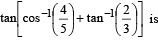Solution: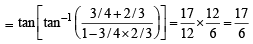QUESTION: 2

### If we consider only the prin ciple values of th e inverse trigonometric functions then the value of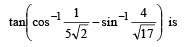Solution: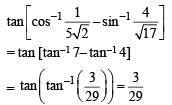QUESTION: 3

### The number of real solutions of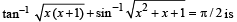Solution: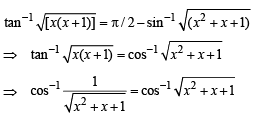⇒ x2 + x+1 = 1⇒ x(x +1) = 0
⇒ x = 0, – 1 are the only real solutions.

QUESTION: 4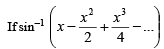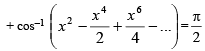for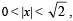then x equals

Solution: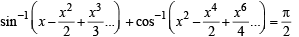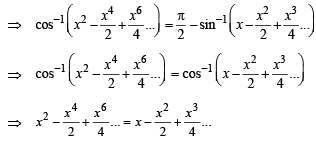On both sides we have G.P. of infinite terms.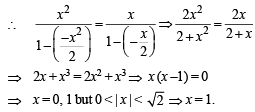QUESTION: 5

If 0 < x < 1, then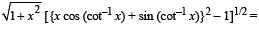Solution: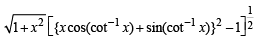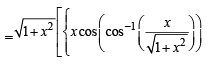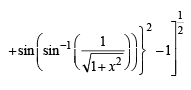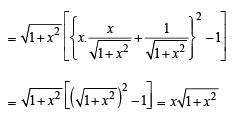QUESTION: 6

The value of x for which sin (cot –1 (1+ x)) = cos (tan–1 x) is

Solution: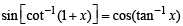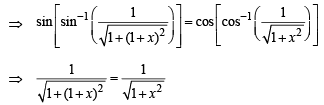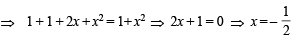QUESTION: 7

The value of cot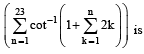Solution: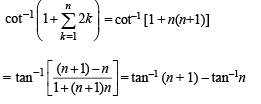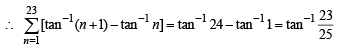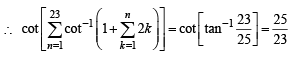QUESTION: 8

The principal value of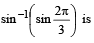Solution:

The principal value of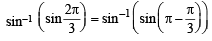= sin –1 (sin π / 3) = π / 3
∴ (d) is the correct answer..

*Multiple options can be correct
QUESTION: 9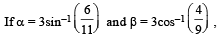where the inverse trigonometric functions take only the principal values, then the correct option(s) is (are)

Solution: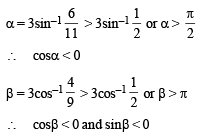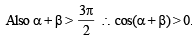Use Code STAYHOME200 and get INR 200 additional OFF Use Coupon Code